# Java基础-泛型机制详解（一）为什么会引入泛型泛

java 泛型这个特性是从 jdk1.5 才开始加入的，因此为了兼容之前的版本，java 泛型的实现采取了 “伪泛型” 的策略，即 java 在语法上支持泛型，但是在编译阶段会进行所谓的 “类型擦除” （Type Erasure），将所有的泛型表示（尖括号中的内容）都替换为具体的类型（其对应的原生态类型），就像完全没有泛型一样。

# 为什么会引入泛型

• 适用于多种数据类型执行相同的代码（代码复用）

``````private static int add(int a, int b){
System.out.println(a + "+" + b + "=" + (a + b));
return a + b;
}

private static float add(float a, float b){
System.out.println(a + "+" + b + "=" + (a + b));
return a + b;
}

private static double add(double a, double b){
System.out.println(a + "+" + b + "=" + (a + b));
return a + b;
}

``````private static <T extends Number> double add (T a，T b){
System.out.println(a + "+" + b + "=" + (a.doubleValue() + b.doubleValue()));
return a.doubleValue() + b.doubleValue();
}

• 泛型中的类型在使用时指定，不需要强制类型转换 （类型安全，编译器会检查类型

``````List list = new ArrayList();

``````List<String> list = new ArrayList<>();
// list 中只能被放 String，不能放其他类型的元素。

# 泛型的基本使用

TIP

## 泛型类

• 从一个简单的泛型类看起：
``````class Point<T>{ // 此处可以随便写标识符号，T 是 type 的简称。
private T var; // var 的类型由 T 指定，即：由尾部决定
public T getVar(){ //返回值的类型由外部决定
return var;
}

public void setVar(T var){ // 设置的类型也由外部决定
this.var = var;
}
}

public class GenericsDemo06{
public static void main(String args[]){
Point<String> p = new Point<String>(); // 里面的 var 类型为 String 类型
p.setVar("it"); // 设置字符串
System.out.println(p.getVar().length); // 取得字符串的长度
}
}

• 多元泛型
``````class Notepad<K, V>{ // 此处制指定了两个泛型类型
private K key; // 此变量的类型由外部决定
private V value; // 此变量的类型由外部决定

public K getKey(){
return this.key;
}
public V getValue(){
return this.value;
}
public void setKey(K key){
this.key = key;
}
public void setValue(V value){
this.value = value;
}
}

public class GenericsDemo09{
public static void main(String args[]){
Notepad<String, Integer> t = null; // 定义两个泛型类型的对象
t = new Notepad<String, Integer>(); // 里面的key为String，value为Integer
t.setKey("汤姆"); // 设置第一个内容
t.setValue(20); // 设置第二个内容
System.out.println("姓名：" + t.getKey()); // 取得信息
System.out.println("姓名：" + t.getValue()); // 取得信息

}
}

## 泛型接口

• 简单的泛型接口
``````interface Info<T>{ // 在接口上定义泛型
public  T getAar(); // 定义抽象方法，抽象方法的返回值就是泛型类型
}

class InfoImpl<T> implements Info<T>{ // 定义泛型借口
private T var; // 定义属性
public Infolmol(T var){ // 通过构造方法设置属性内容
this.setVar(var);
}
public void setVar(T var){
this.var = var;
}
public T getVar(){
return this.var;
}
}

public class GennericsDemo24{
public static void main(String args[]){
Info<String> i = null; // 声明接口对象
i = new InfoImpl<String>("汤姆"); // 通过子类实例化对象
System.out.println("内容：" + i.getVar());
}
}

## 泛型方法

• 泛型方法，是在调用方法的时候指明泛型的具体类型。
• 定义泛型方法语法格式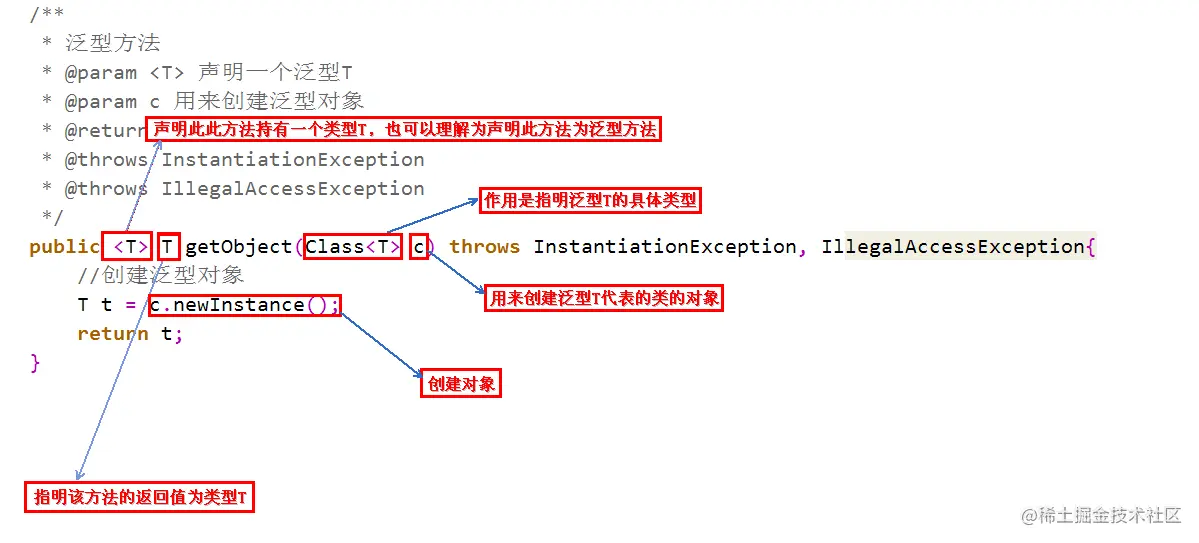• 调用泛型方法语法格式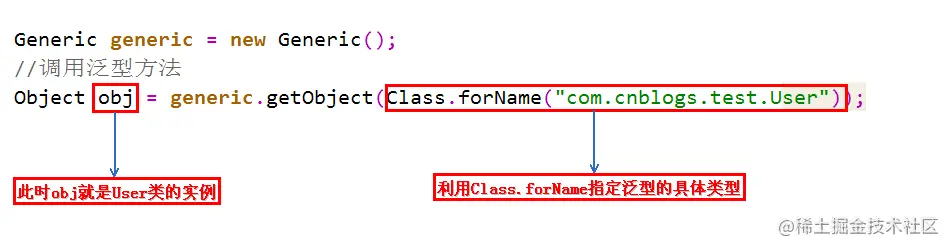Class 的作用就是指明泛型的具体类型，而 Class 类型的变量 c，可以用来创建泛型类的对象。

**为什么要使用泛型方法呢？**因为泛型类要在实例化的时候就指明类型，如果想换一种类型，不得不重新 new 一次，可能不够灵活；而泛型方法可以在调用的时候指明类型，更加灵活。

## 泛型的上下限

• 先看下如下的代码，很明显是会报错的。
``````class A{}

Class B extends A{}

// 如下两个方法不会报错
public static void funA(A a){
// ....
}
public static void funB(B b){
funA(b);
// ....
}

// 如下 funD 方法会报错
public static void funC(List<A> listA){
// ....
}
public static void funD(List<B> listB){
/* 未解析的编译问题:类型测试中的方法doPrint(List<A>)不适用于参数(List<B>)
* Unresolved compilation problem: The method doPrint(List<A>) in the type test is not applicable for the arguments (List<B>)*/
// funC(listB);
// ....
}

``````public static void funC(? <extends A> listA){
// ....
}
public static void funD(List<B> listB){
funC(listB); // ok
// ....
}

• 泛型上下限的引入

``````class Info<T extends Number>{ // 此处泛型只能是数字类型
private T var; // 定义泛型变量
public void setVar(T var){
this.var = var;
}
public T getVar(){
return this.var;
}
public String toString(){ // 直接打印
return this.var.toString();
}
}

public class demo1{
public static void main(String args[]){
Info<Integer> il = new Info<Integer>(); // 声明 Integer 的泛型对象
}
}

``````class Info<T>{
private T var; // 定义泛型变量
public void setVar(T var){
this.var = var;
}
public T getVar(){
return this.var;
}
public String toString(){ // 直接打印
return this.var.toString();
}
}
public class GenericsDemo21{
public static void main(String args[]){
Info<String> i1 = new Info<String>(); // 声明 String 的泛型对象
Info<Object> i2 = new Info<Object>(); // 声明 Object 的泛型对象
i1.setVar("hello");
i2.setVar(new Object());
fun(i1);
fun(i2);
}

public static void fun(Info<? super String> temp){ // 只能接收 String 或 Object 类型的泛型，String 类的父类只有 Object 类
System.out.println(temp + ",");
}
}

• 小结
``````<?> 无限制通配符
<? extends E> extends 关键字声明了类型的上界，表示参数化的类型可能是所指定的类型，或者是此类型的子类。
<? super E> super 关键字声明了类型的下界，表示参数化的类型可能是指定的类型，或者是此类型的父类。

/* 使用原则《Effictive Java》
* 为了获得最大限度的灵活性，要在表示 生产者或者消费者 的输入参数上使用通配符，使用的规则就是：生产者有上限，消费者有下限。 */
1.如果参数化类型表示一个 T 的生产者，使用 <? extends T>;
2.如果它表示一个 T 的消费者，就使用 <? super T>;
3.如果即是生产又是消费，那么使用通配符就没有什么意义了，因为你需要的是精确的参数类型。

• 在看一个实际例子，加深印象。
``````private <E extends Comparable<? super E>> E max(List<? extends E> el){
if(el == null){
return null;
}

// 迭代器返回的元素属于 E 的某个子类型。
Iterator<? extends  E> iterator = el.iterator();
E result = iterator.next();
while (iterator.hasNext()){
E next = iterator.next();
if(next.compareTo(result) > 0){
result = next;
}
}
return result;
}

• 要进行比较，所以 E 需要是可比较的类，因此需要 extends Comparable<...> (注意这里不要和继承的 extends 搞混了，不一样)。
• Comparable<? super E> 要对 E 进行比较，即 E 的消费者，所以需要用 super。
• 而参数 List<? extends E> 表示要操作的数据是 E 的子类的列表，指定上限，这样容器才够大。

## 多个限制

``````public class Client{
// 工资低于 2500 元的上班族并且独立站立的乘客车票打8折
public static <T extends Staft & Passenger> void discount(T t){
if(t.getSalary() < 2500 && t.isStanding()){
System.out.prinln("恭喜你！您的车票打八折！");
}
}
public static void main(String[] args){
discount(new Me());
}
}

## 泛型数组

``````List<String>[] list1 = new ArrayList<String>; // 编译错误，非法创建。
List<String>[] list2 = new ArrayList<?>; // 编译错误，需要强制转换类型
List<String>[] list3 = (List<String>[])new ArrayList<?>; // ok，但是会有警告
List<?>[] list4 = new ArrayList<String>; // 编译错误，非法创建
List<?>[] list5 = new ArrayList<?>; // ok
List<String>[] list6 = new ArrayList; // ok，但是会有警告

• 讨巧的使用场景
``````public class GenericsDemo{
public static void main(String args[]){
Integer i[] = fun1(1,2,3,4,5,6); // 返回泛型数组
fun2(i);
}

public static<T> T[] fun1(T... arg){ // 接收可变参数
return arg; // 返回泛型数组
}

public static<T> void fun2(T param[]){ // 输出
System.out.println("接收泛型数组：");
for(T t : param){
System.out.print(t + "、");
}
}
}

• 合理使用
``````puplic ArrayWithTypeToken(Class<T> type, int size){
array = (T[])Array.newInstance(type, size);
}

# 深入理解泛型

## 如何理解 Java 中的泛型是伪泛型？泛型中类型擦除？

java 泛型这个特性是从 JDK1.5 才开始加入的，因此为了兼容之前的版本，java 泛型的实现采取了**“伪泛型”的策略，即 Java 在语法上支持泛型，但是在编译阶段会进行所谓的“类型擦除”**（Type Erasure），将所有的泛型表示（尖括号中的内容）都替换为具体的类型（其对应的原生态类型），就像完全没有泛型一样。理解类型擦除对于用好泛型是很有帮助对的，尤其是一些看起来“疑难杂症”的问题，弄明白了类型擦除也就迎刃而解了。

• 消除类型参数声明，即删除 <> 及其包围的部分。
• 根据类型参数的上下界推断并替换所有的类型参数为原生态类型：如果类型参数是无限制通配符或没有上下界限定则替换为 Onject，如果存在上下界限定则根据子类替换原则取类型参数左边限定类型（即父类）。
• 为了保证类型安全，必要时插入强制类型转换代码。
• 自动产生“桥接方法”以保证擦除类型后的代码依然具有泛型的“多态性”。

### 那么如何进行擦除的呢？

• 擦除类定义中的类型参数 -无限制类型擦除。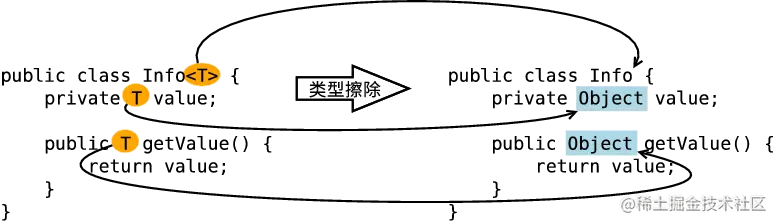• 擦除类定义中的类型参数 -有限制类型擦除。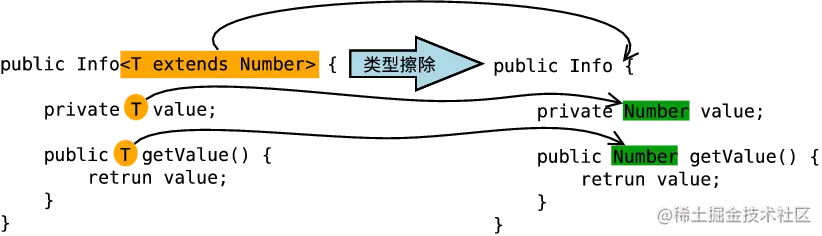• 擦除方法中定义的类型参数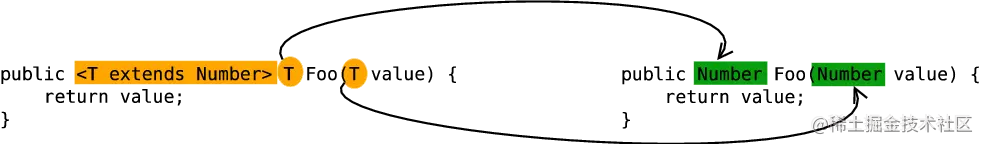## 如何证明类型的擦除呢？

• 元素类型相等
``````public class Test{
public static void main(String[] args){
ArrayList<String> list1 = new ArrayList<String>();
ArrayList<Integer> list2 = new ArrayList<Integer>();
System.out.println(list1.getClass() == list2.getClass()); // true
}
}

``````在这个例子中，我们定义了两个 ArrayList 数组，不过一个是 ArrayList<String> 泛型类型，只能存储字符串；一个是 ArrayList<Integer> 泛型类型，只能存储整数；最后，我们通过 list1 和 list2 对象的 getClass() 方法获取他们的类的信息，最后发现结果为 true。

• 通过反射添加其他类型元素
``````public class Test {

public static void main(String[] args) throws IllegalAccessException, IllegalArgumentException,
InvocationTargetException, NoSuchMethodException, SecurityException {
ArrayList<Integer> list = new ArrayList<>();

for (int i = 0; i < list.size(); i++) {
System.out.println(list.get(i));
}

}
}

``````在程序中定义了一个 ArrayList 泛型类型实例化为 Integer 对象，如果直接调用 add() 方法，那么只能存储整数数据，不过当我们利用反射调用 add() 方法的时候，却可以存储字符串，这说明了 Integer 泛型实例在编译之后被擦除掉了，只保留了原始类型。

## 如何理解类型擦除后保留的原始类型？

• 原始类型 Object
``````public class Pair<T> {
private T value;

public T getValue() {
return value;
}

public void setValue(T value) {
this.value = value;
}
}

Pair 的原始类型为：

``````public Pair{
private Object value;

public Object getValue(){
return value;
}

public void setValue(Object value){
this.value = value;
}
}

``````public class Pair<T extends Comparable>{}

• 在不指定泛型的情况下，泛型变量的类型为该方法中的几种类型的同一父类的最小级，直到 Object.
• 在指定泛型的情况下，该方法的几种类型必须是该泛型的实例的类型或者其子类。
``````public class Test{
public static void mian(String[] args){
/* 不指定泛型的时候 */
int i = Test.add(1, 2); // 这两个参数都是 Integer，所以 T 为 Integer 类型。
Number f = Test.add(1, 1.2); // 这两个参数一个是 Integer，一个是 Float，所以取同一父类的最小级，即 Number。
Object o = Test.add(1, "abc");

/* 指定泛型的时候 */
int a = Test.<Integer>add(1, 2); // 指定了 Integer,所以只能为 Integer 类型或者其子类。
int b = Test.<Integer>add(1, 1.2); // 编译错误，指定了 Integer，不能为 Float。
Number c = Test.<Number>add(1, 2.2); // 指定为 Number，所以可以为 Integer 和 Float。
}

// 这是一个简单的泛型方法
public static <T> T add(T x, T y){
return y;
}
}

• Object泛型
``````public static main(String[] args){
ArrayList list = new ArrayList();

}

## 如何理解泛型的编译期检查？

Java 编译器是通过检查代码中泛型的类型，然后在进行类型擦除，在进行编译。

``````public static void main(String[] args){
ArrayList<String> list = new ArrayList<String>();
}

``````ArrayList list = new ArrayList();

``````ArrayList<String> list = new ArrayList<String>();

``````ArrayList<String> list1 = new ArrayList(); // 第一种情况
ArrayList list2 = new ArrayList<String>(); // 第二种情况

``````public class Test{
public static void main(String[] args){
ArrayList<String> list1 = new ArrayList();
String str1 = list1.get(0); // 返回类型就是 String

ArrayList list2 = new ArrayList<String>();
Object object = list2.get(0); // 返回类型就是 Object

new ArrayList<String>().add("11"); // 编译通过
new ArrayList<String>().add(22); // 编译错误

String str2 = new ArrayList<String>.get(0); // 返回类型就是 String
}
}

``````ArrayList<String> list1 = new ArrayList<Object>(); // 编译错误
ArrayList<Object> list2 = new ArrayList<String>(); // 编译错误

• 我们先看第一种情况，将第一种情况扩展成下面的形式：
``````ArrayList<Object> list1 = new ArrayList<Object>();
ArrayList<String> list2 = list1; //编译错误

• 再看第二种情况，将第二种情况扩展成下面的形式：
``````ArrayList<String> list1 = new ArrayList<String>();